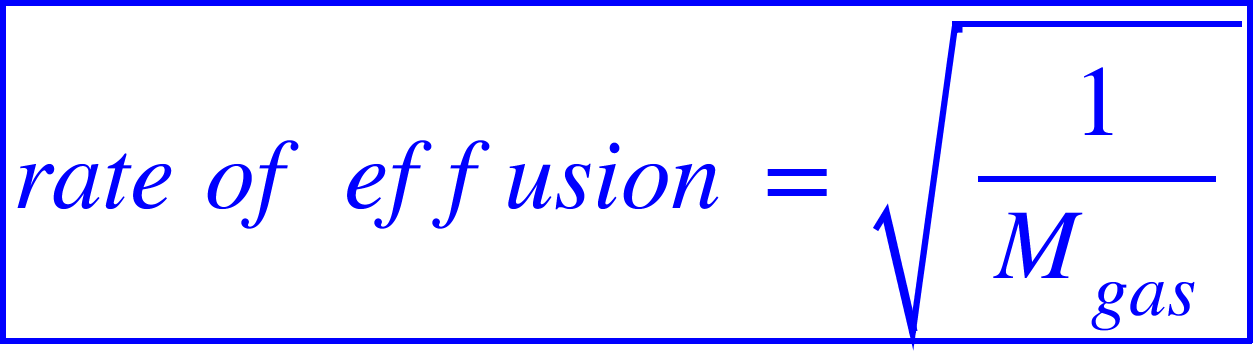# Problem: A mixture of gaseous disulfur difluoride, dinitrogen tetrafluoride, and sulfur tetrafluoride is placed in an effusion apparatus.(a) Rank the gases in order of increasing effusion rate.

###### FREE Expert Solution

Effusion is a process where a gas escapes from a container through a very tiny hole.

The rate of effusion of a gas decreases as its molar mass increases.

This is represented by the following relation:This means:

• Higher the molar mass of a gas, lower the rate of effusion.

91% (404 ratings)###### Problem Details

A mixture of gaseous disulfur difluoride, dinitrogen tetrafluoride, and sulfur tetrafluoride is placed in an effusion apparatus.

(a) Rank the gases in order of increasing effusion rate.

Frequently Asked Questions

What scientific concept do you need to know in order to solve this problem?

Our tutors have indicated that to solve this problem you will need to apply the Effusion concept. You can view video lessons to learn Effusion. Or if you need more Effusion practice, you can also practice Effusion practice problems.

What textbook is this problem found in?

Our data indicates that this problem or a close variation was asked in Chemistry: The Molecular Nature of Matter and Change - Silberberg 8th Edition. You can also practice Chemistry: The Molecular Nature of Matter and Change - Silberberg 8th Edition practice problems.